# Numerical Analysis of Film Cooling Shield Formed by Confined Jet Discharging on a Flat Plate

Numerical Analysis of Film Cooling Shield Formed by Confined Jet Discharging on a Flat Plate

Mohammed Al-Hemyari Mohammad O. HamdanMehmet F. Orhan

Mechanical Engineering Department, College of Engineering, American University of Sharjah, Sharjah 26666, United Arab Emirates

Corresponding Author Email:
mhamdan@aus.edu
Page:
327-333
|
DOI:
https://doi.org/10.18280/ijht.370139
13 February 2019
|
Accepted:
15 March 2019
|
Published:
31 March 2019
| Citation

OPEN ACCESS

Abstract:

The effects of centrifugal force and thermal conductivity on the effectiveness of a film cooling shield are investigated in this study. A confined jet with 90 degree angle is used, to inject cooling fluid into hot steam, to form a film cooling shield that protect a flat plate. Film cooling is modelled in 2D using ANSYS Fluent commercial computation fluid dynamic tool. The RNG k-ε turbulence model with enhanced wall function (EWF) is selected to capture the low-Reynolds number effects near the wall. The selected turbulence model has showed better prediction of the adiabatic film cooling effectiveness accuracy (AFCE) compared to other turbulence models. The results show that centrifugal force alters the flow field and affects the film cooling shield attachment to the flat plate. A clear drop in the AFCE is observed when positive centrifugal force acts perpendicular on the confined jet, which causes overheating in the vicinity of the jet. The effect of wall thermal conductivity on film cooling effectiveness FCE is reported using different thermal conductivity ratios between wall and fluid; mainly, 1, 10, 100, 1,000 and 10,000. The results show that thermal conductivity ratios less than 1 have almost no effect on FCE while high thermal conductivity ratios deteriorate the FCE in the vicinity of the jet.

Keywords:

1. Introduction

Thermal management is vital for many engineering applications such as gas turbines, electronics cooling and power generation. Thermal management of a gas turbine can directly impact its performance. For instance, proper cooling of gas turbine blades allows higher gas turbine inlet temperature, which leads to higher turbine’s power output and thermal efficiency . Advanced gas turbine engines operate at temperatures higher than 1,200℃ [2-3]. These high inlet temperatures cause hot spot formation and elevate wall thermal stresses on turbine's blade, which reduce the turbine blade life. To assure realistic turbine blade life, the variation on the blade wall temperature must be limited . Remarkable work has been done, in the fields of material and thermal science, to increase turbine maximum allowable inlet gas temperature. Since the development of aircraft gas turbine in 1941, the average rate of increasing the maximum allowable inlet temperature using cooling techniques is around 20℃ every year which is more than double the rate achieved by material scientist . Most of today’s advanced gas turbines utilizes cooling techniques in their gas turbine blade. Cooling techniques are classified into internal cooling methods such as internal jet impingement [6-10] and external methods such as transpiration cooling  and film cooling [12-13].

Gas turbine film cooling is achieved by injecting cool air between the external hot gases and the blade wall. The cool air is bled from the compressor and is used to form a film cooling shield around the blade to shield it from surrounding hot gases. A very wide list of parameters that affect the performance of the film cooling are reported in the literature. Researchers have experimentally and numerically investigated the performance of film cooling parameters. The film cooling performance is reported using film cooling effectiveness (FCE) which is defined as follow,

$η=(T_h-T_w)/(T_h-T_c)$ (1)

where, η, $T_h$, $T_w$ and $T_c$ are the film cooling effectiveness, mainstream hot gas temperature, turbine's blade wall temperature and coolant gas temperature, respectively.

The parameters that effect the film cooling can be classified into two main groups; flow parameters and geometrical parameters. Flow parameters are generally reported in terms of blowing ratio (M) [14-17], density ratio (DR) [18-19] and turbulence intensity (I) [20-21]; while geometrical parameters are reported in terms of injection angle (α) [17, 22-23], hole shape [24-26], number of holes, jet delivery channel length and hole to hole spacing . All these parameters are interrelated, and their combinations affect the performance of the adiabatic film effectiveness [28-29].

Few studies on the other hand are available in literature that have explored the impact of centrifugal force on film cooling. The experimental difficulty has limited number of these studies. A high impact of centrifugal force on film cooling effectiveness is expected since centrifugal force will act as a body force that would alter the flow direction and will affect the film attachment to the wall. The magnitude of the centrifugal force is directly related to engine angular speed, which often operates around 1000 RPM. Zhu et al. experimentally and numerically reported the performance of FCE at low angular speed . For a rotor with an angular speed of 300 RPM, their results have showed that the centrifugal force effects on the pressure side of the turbine blade are more significant than on the suction side. They have also reported that the span wise area averaged FCE drops as flow moves towards the blade tip. Alzurfi et al. numerically studied the effect of centrifugal force on a low speed . They reported that the low FCE at the pressure side is mainly due to a favourable pressure driving the coolant away from the blade wall, while an adverse pressure gradient is observed at the suction side forcing the coolant to stay attached to the wall. These different pressure gradients have caused flow detachment and attachment, which occurred at different blowing ratios and centrifugal effects on both sides.

Numerical studies often report the effectiveness of film cooling as adiabatic film cooling effectiveness (AFCE) since most of the turbine blades are made of a low thermal conductivity materials or super alloys. To incorporate the effect of the wall thermal conductivity, conjugate heat transfer is used. Rigby and Lepicovsky studied the capability of conjugate heat transfer to capture flow and thermal fields of an internally cooled configuration . They reported that the numerical results implementing the conjugate heat transfer have successfully captured the experimentally obtained flow and thermal fields. Silieti et al. reported that different turbulence models , mainly Reynolds normalization group (RNG) k–ε, the realizable k–ε and the Reynolds transport turbulence models, can be used to numerically capture the conjugate heat transfer effect. They also reported that blade walls made of steel show a 10% deficiency in FCE compared to the AFCE.

In this study, a numerical model in 2D is used to study the effect of centrifugal force and wall thermal conductivity on film cooling shield. This shield is formed by a confined jet that is discharging a cooling film to a hot stream flow. The study presents local AFCE for various centrifugal forces between -100,000 and 100,000 m/s^2. Also, the study reports the local FCE results with different thermal conductivity ratios ranging from 1 to 10,000.

2. Problem Formulation

The book size will be in A4 (8.27 inches x 11.69 inches). Do not change the current page settings when you use the template.

The number of pages for the manuscript must be no more than ten, including all the sections. Please make sure that the whole text ends on an even page. Please do not insert page numbers. Please do not use the Headers or the Footers because they are reserved for the technical editing by editors.

The schematic diagram of the computational domain is show in Figure 1, where the two inlets are referred to the inlets of hot mainstream and the cool confined jet flow. The hot and cool flow mixes and leave from one outlet. And, the hot mainstream fluid flows from left to right. Also, the coolant fluid enters from the bottom side of the plate. The dimensions are selected based on the experimental work of O'Malley . The confined jet width is D=40 mm and is located 4D from the downstream of the hot gas inlet. The coolant channel height is 3.5D, the mainstream inlet height is 6D and the size of the computational domain is 12D×57D. The outlet is in the far end of the hot mainstream flow (located at the right side of Figure 1) and is treated as pressure outlet with zero gage pressure. All the walls are set to no-slip and adiabatic condition.

Air is used as the working fluid for the mainstream and the cooling jet. Air is treated as an incompressible ideal gas. Air density is calculated using the ideal gas equation while the specific heat, thermal conductivity and dynamic viscosity are obtained using Eqns. (2), (3) and (4), respectively .

Specific heat, $C_p (J/kg∙K)$,

$C_p=9.08×10^{-11} T^4-4.81×10^{-7} T^3+8.07×10^{-4} T^2-0.321 T+1.05×10^3$ (2)

Thermal conductivity, $k_c (W/m∙K)$,

$k_c=7.99×10^{-12} T^3-2.40×10^{-8} T^2+8.30×10^{-5} T+2.88×10^{-3}$ (3)

Dynamic viscosity, $μ ( kg/m∙s$),

$μ=1.70×10^{-14} T^3-4.04×10^{-11} T^2+6.85×10^{-8} T+1.06×10^{-6}$ (4)

where, T is fluid temperature in kelvin.

## 1.png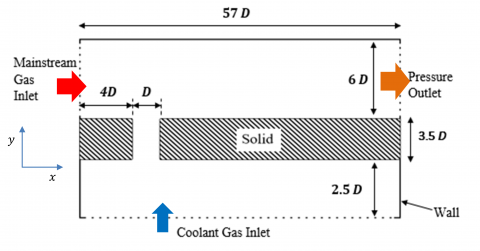Figure 1. Schematic diagram of computational domain

The adiabatic film cooling effectiveness (AFCE) is used to evaluate the performance of film cooling under different parameters, which is calculated as shown in Eq. 5.

$η_ad=(T_{hot}-T_{ad-wall})/(T_{hot}-T_{cold})$  (5)

where, subscript (ad) refers to adiabatic conditions at the wall of the jet.

The centrifugal force effect is explored using the centripetal acceleration at the hole specific location and turbine rotational speed. The centripetal acceleration is defined as follow,

$a_c=ω_t^2*r$ (6)

where, $a_c$, $ωt$ and r are the centripetal acceleration, angular acceleration and location of the hole from axis of rotation, respectively.

The effect of solid wall thermal conductivity is investigated by evaluating various thermal conductivity ratios $K_R$. Thermal conductivity ratio is defined in Eq. 7 as the ratio of thermal conductivities of the solid wall to the coolant air at 360 ℃.

$K_R=k_w/k_{c at 360C} =k_w/0.03$ (7)

3. Numerical Modelling

A structured mesh is generated using a commercial software from Pointwise with bias fine mesh near the wall. Since cells near to the wall are required to have a $y^+$ of 1.0 by the enhanced wall function (EWF) treatment, a near-wall mesh guidelines are employed where the height of first cell closest to the wall is calculated as 0.00017 D. This was enough to have a $y^+$ value less than unity. Figure 2 shows the generated mesh with a closer look near the confined perpendicular jet.

## 2.pngFigure 2. Computational domain showing the mesh generated with a close look near the perpendicular jet

The governing equations used in this study are conservation of mass, momentum and energy, which are listed below in index notation,

$(∂(ρu_i))/(∂x_i)=0$ (8)

$(∂(ρu_j u_i))/(∂x_j )=∂P/(∂x_j )+∂/(∂x_j ) [μ((∂u_i)/(∂x_j )+(∂u_j)/(∂x_i ))-ρ(u ́_i u ́_j ) ̅ ]$ (9)

$(∂(ρu_j T))/(∂x_j )=∂/(∂x_j ) [μ/Pr (∂T/(∂x_j ))-ρ(T ́_i u ́_j ) ̅ ]$ (10)

where, $u_i$, T and P are the mean velocity, temperature, and pressure, respectively. The acute accent (´) represents the fluctuations in the flow variable. The time-averaged component in the momentum and energy equations are modelled using the Boussinesq hypothesis and the simple eddy diffusivity model as shown in the following equations, respectively,

$ρ(u ́_i u ́_j ) ̅=μ_t ((∂u_i)/(∂x_j )+(∂u_j)/(∂x_i ))-2/3 ρkδ_{ij}$ (11)

$ρ(T ́_i u ́_j ) ̅=-μ_t/Pr_t ∂T/(∂x_j )$ (12)

where, $μ_t$, k and $Pr_t$ are the turbulent viscosity, turbulent kinetic energy and turbulent Prandtl number, respectively.

Turbulence models are used to solve Eqns. 8, 9 and 10 by estimating different turbulent parameters that appear in Eqns. 11 and 12. The -ε, k-ω and the Menter's Shear Stress Transport (SST) turbulence models are tested to evaluate the appropriateness of these turbulence models for current problem. For all k-ε turbulence models, EWF treatment is used for near-wall modelling. However, for k-ω and SST models, near-wall modelling is not needed since these models include blending functions in the near wall region that are a function of wall distance .

The governing equations are discretized using the second-order upwind scheme and the pressure-velocity coupling is resolved using the semi implicit method for pressure linked equations consistent (SIMPLEC). The numerical solution convergence is achieved when the temperature residual reaches less than $10^{-9}$ and the velocities and continuity residuals reach less than $10^{-6}$.

4. Results and Discussion

In the computational analysis, three meshes are generated and tested, namely the coarse mesh with 145,000 elements, the moderate mesh with 280,000 elements and the fine mesh with 500,000 elements. The RNG k-ε with EWF turbulence model is selected for the grid independence study. The mesh independent study is presented in Figure 3. It can be observed from the figure that there is no significant difference between the results for the fine and moderate mesh sizes. Both have a relative error of 1.7 % in comparison to the numerical results of Bayraktar and Yilmaz . Meanwhile, the coarse mesh had a relative error of about 4.5 %. Therefore, the moderate size mesh with 280,000 elements is selected in this study.

## 3.pngFigure 3. Mesh independent study and comparison to literature 

The validity of current CFD model has been tested against experimental work as show in Figure 4a and against numerical work as shown in Figure 4b. The velocity profile obtained by O’Malley  is plotted versus current CFD work using RNG k-ε with EWF turbulence model. As shown in the figure, current model is capable to capture reverse flow near the wall at distance x/D=0.3 which is reported by O’Malley  experimental measurements. To select an optimum turbulence model in predicting the AFCE that match published results, an assessment analysis of different turbulence models is carried out as shown in Figure 4b. As illustrated in figure 4b, all the models show the same trend in the AFCE curves. Comparing with the numerical results in , the RNG k-ε model shows the best accuracy with an overall deviation of 1.7 %. This is followed by the standard k-ε model with a deviation of 6.21 %. The other turbulence models show a higher deviation relative to the k-ε models. For instance, the standard k-ω results in a deviation of 26.4 %, the SST k-ω shows 26.84 %, while the SST model yields 27.6 % deviation. As evident in Eq. 2, the reported AFCE depends only on the wall temperature and the accuracy of the turbulence model depends on how the boundary layer of film cooling is treated. The RNG k-ε model with EWF reports the best accuracy, since the effective turbulence viscosity includes the low-Reynolds number effects near the wall. Therefore, in this study, the RNG k-ε model is adopted as the turbulence model to simulate and understand the effect of both centrifugal force and blade thermal conductivity on FCE.

## 4-1.png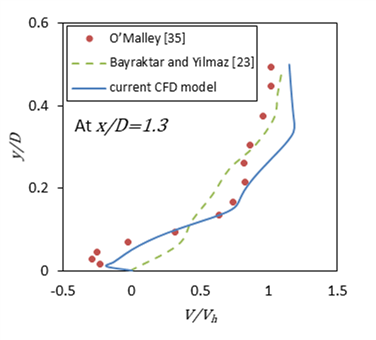## 4-2.pngFigure 4. Results validation (a) velocity profile at distance x/D=1.3 based on experimental work  (b) turbulence model selection and comparison based on numerical work 

4.1 Centrifugal force

Centrifugal force is a body force that alters the flow field and affects the film cooling shield attachment to the solid wall. From Eq. 6, it is anticipated that the centrifugal force has maximum effect on the tip cap holes shown in Figure 5 and identified in zone 3. Modern macro-gas turbine engines can operate at rotational speeds of about 15,000 RPM. This high rotational speed produces a very high centripetal acceleration at the tip that can reach up to $2.7×10^6$ $m/s^2$ on a two meters diameter turbine. From Eq. 6, the centrifugal force increases with the increase of the turbine angular velocity. Hence the centripetal acceleration is calculate using Eq. 6 and this value has been used as body force in the numerical modelling. A various centrifugal force between -100,000 and 100,000 m/s^2 are tested and were enforced in the positive y-direction of the computation domain. The positive sign means the centripetal acceleration is moving fluid far from the flat plate while negative sign means the centripetal acceleration is doing the opposite.

## 5.jpg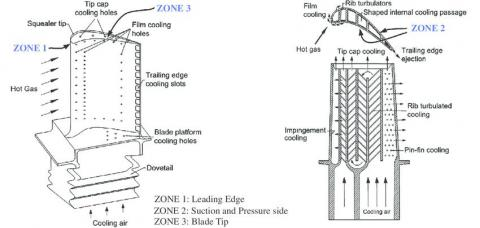Figure 5. A cooled turbine blade configuration 

Figure 6 shows the AFCE curves at different centripetal accelerations using a blowing ratio ($M=ρ_c V_c/ρ_h V_h$) of 0.1, an injection angle (α) of 90° and a density ratio ($DR=ρ_c/ρ_h$) of 2.0. The subscripts c refers to coolant while h refers to hot mainstream. Negative centripetal acceleration refers to negative y-direction, while positive centripetal acceleration to positive y-direction. Figure 6 shows that, as the centripetal acceleration increases the local AFCE drops, which indicates that the overall AFCE will drop as well. In general, centrifugal forces lift the film cooling layer away from the wall and cause the AFCE to drop significantly. Hence, centrifugal forces detach the cooling film and expose the blade to the mainstream high temperature, which leads to overheating at the wall. In gas turbine, overheating at the blade tip is considered one of the main reasons for turbine blades thermal stress failure . To prevent this thermal stress failure at the turbine blade tip, a non-uniform distribution, more biased toward the tip, of the film cooling holes must be used . From Figure 6, it is clear that centripetal acceleration is directly impacting the AFCE. The figure shows that for centripetal absolute value higher than 10,000 $m/s^2$ the impact of AFCE is dramatic and needs to be considered in film cooling design.

## 6.pngFigure 6. AFCE curves for different centripetal accelerations

4.2 Effect of wall thermal conductivity

In order to study the impact of flat plate thermal conductivity, conjugate heat transfer needs to be solved at the wall, hence adiabatic wall condition is not appropriate to use. In this part of the study, the wall is not adiabatic and hence FCE is reported instead of AFCE. As listed in Table 1, various$K_R$ values are used in this study.

Table 1. Thermal conductivity values of the blade wall

 $K_R$ $k_w (W/m.K)​$ 1 0.03 100 3 1,000 30 10,000 300

The effect of different thermal conductivity ratios is shown in Figure 7. As shown in the figure, low thermal conductivity material with $K_R$=1.0 shows no major difference when compared to the AFCE values under same conditions (i.e. M=0.1, α=90° and DR=1.2). As evident from the figure, increasing the thermal conductivity ratio $K_R$ leads to a decrease in the FCE directly after the hole and an increase in FCE after certain distance downstream of the hole. The reduction in FCE near the hole is due to the increase in the coolant temperature, which occur due to the increase in heat conduction between the mainstream and cooling fluid. Increasing wall thermal conductivity allows better spreading of heat transfer across the flat plate which flattens the temperature distribution at the wall. The increase in the cooling fluid temperature reduces the effect of the film cooling shield. On the other hand, as film cooling flow marches downstream, the film cooling shield effect deteriorates due to flow mixing and convective heat transfer. Therefore, the FCE raises with the increase in thermal conductivity. This rise is driven by the enhanced wall ability to conduct more heat to the coolant plenum causing a reduction in the wall temperature. In short, FCE increases in the beginning near the hole and then decreases far from the hole.

To properly estimate the effect of wall thermal conductivity, one needs to calculate the overall FCE that is defined as the area-weighted average of FCE. For current 2D problem, the overall FCE is calculated based on the distance between holes. If the spacing between two consecutive holes is set to be 5D the drop in overall FCE is around 6% for $K_R$=100, however, at $K_R$=10,000 the drop in overall FCE is about 45 %.

The thermal conductivity ratio has direct effect on wall thermal stresses. A higher thermal conductivity indicates a less temperature gradient at the wall. Lower temperature gradient is desired to reduce thermal stresses within the wall. Hence, one needs to optimize the wall thermal conductivity based on the maximum temperature gradient that the material can withstand. This shows that there is a room to improve the material selection, so it can withstand high temperature and high temperature gradients.

Figure 8 shows how the wall temperature gradient varies for two thermal conductivity ratios. At $K_R=1$, the coolant plenum experiences no heating from the mainstream gas, before and after the film cooling effect. However, at $K_R$=10,000 the plenum wall temperature near the film cooling hole is lower, which increases the temperature of the coolant and hence reduces FCE. As shown in Figure 8, a wall with $K_R$ of 1 (Figure 8a) has lower overall average temperature compared to a wall with $K_R$ of 10,000 (Figure 8b). Also, as shown in Figure 8, a wall with  $K_R$ of 1 has higher thermal temperature gradient compared to a wall with $K_R$ of 10,000. Higher $K_R$ is desired to avoid high temperature gradient in the wall and lower is desired to improve the effectiveness of film cooling. An optimization between wall temperature gradient and wall maximum temperature which depends on holes shape, number and angle.

## 7.png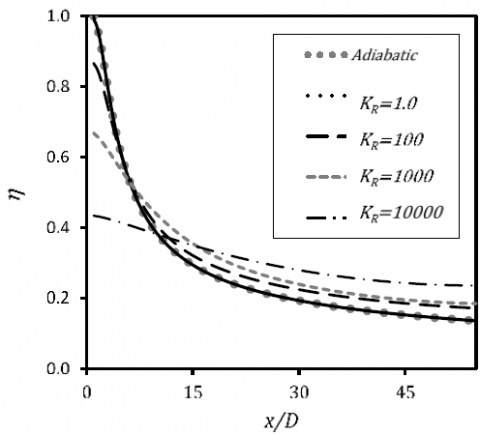Figure 7. FCE under conjugate heat transfer for different thermal conductivity ratios $K_R$

## 8.jpg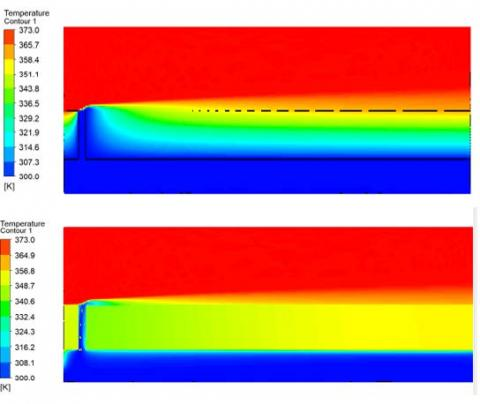Figure 8. Wall temperature gradient for (a) $K_R$=1.0 and (b) $K_R$=10,000

5. Conclusions

In this 2D numerical study, the effect of centrifugal force and wall thermal conductivity on film cooling performance are examined using ANSYS FLUENT. The RNG k-ε turbulence model with EWF has shown better capability compared to k-ω and SST models. Centrifugal force alters the flow field and affects the film cooling shield attachment to the flat plate causing the AFCE to fall under positive centrifugal accelerations, which causes wall overheating. Finally, conjugate heat transfer analysis has been used to understand the effect of thermal conductivity on FCE. Thermal conductivity ratio of one ($K_R=1$) has produced similar results as the adiabatic wall case. High thermal conductivity ratios reduce film cooling shielding effect near the hole and increase it far away from the hole. This work can be extended by testing the effect of multiple jet with different jet angle.

Nomenclature
 $a_c$ centripetal acceleration, [m/s2] AFCE adiabatic film cooling effectiveness $C_p$ specific heat, [J/kg.K] D slot diameter, [m] DR density ratio of coolant to mainstream gas, [-] EWF enhanced wall function FCE film cooling effectiveness $K_R$ thermal conductivity ratio, [-] k turbulence kinetic energy, [m2/s2] $k_c$ thermal conductivity, [W/m.k] L mainstream entrances length, [m] M blowing ratio, [-] P pressure, [kPa] Pr Prandtl number, [-] RSM response surface method RNG Reynolds normalization group RPM revolution per minutes SST Menter’s shear stress transport T temperature, [K] V velocity, [m/s] VR velocity ratio of coolant to mainstream gas, [-] x distance in the mainstream gas flow direction, [m] y distance in the hole normal direction, [m]

Greek symbols

 α injection angle. [deg] $\varepsilon$ turbulence dissipation rate, [ J/kg.s ] $\eta$ film cooling effectiveness, [-] $\mu$ dynamic viscosity, [kg/m.s ] $\rho$ density, [ kg/m3 ] $\omega$ turbulence specific dissipation rate, [1/s ]

Subscripts

 ad adiabatic av average c coolant jet h mainstream gas w wall t turbulent

References

 Çengel Y, Boles M. (2015). Thermodynamics: An engineering approach. 8th Edition. McGraw-Hill Education, New York.

 Straub D, Sidwell T, Casleton K, Chien S, Chyu M. (2012). High temperature film cooling test facility and preliminary test. ASME Turbo Expo, Copenhagen, Denmark, pp. 1661-1671. http://dx.doi.org/10.1115/GT2012-69767

 Chyu M, Siw S. (2013). Recent advances of internal cooling techniques for gas turbine airfoils. Journal of Thermal Science and Engineering Applications 5(2): 021008. http://dx.doi.org/10.1115/1.4023829

 Rezazadeh R, Alizadeh M, Alireza F, Khaledi H. (2013). Turbine blade temperature calculation and life estimation - a sensitivity analysis. Propulsion and Power Research 2(2): 148-161. http://dx.doi.org/10.1016/j.jppr.2013.04.004

 Lakshminarayana B. (1995). Fluid dynamics and heat transfer of turbomachinery. John Wiley & Sons. http://dx.doi.org/10.1002/9780470172629

 Elnajjar E, Hamdan M, Haik Y. (2013). Experimental investigation of internal channel cooling via jet impingement. FDMP: Fluid Dynamics & Materials Processing 9(1): 77-89.

 Hamdan M, Hayek A. (2013). Effect of cavity size on confined slot jet impingement cooling. ASME 2013 Heat Transfer Summer Conference, Minneapolis, USA, pp. V002T04A013-V002T04A013. http://dx.doi.org/10.1115/HT2013-17026

 Hamdan M, Elnajjar E, Haik Y. (2011). Measurement and modeling of confined jet discharged tangentially on a concave semicylindrical hot surface. ASME Journal of Heat Transfer 133(12): 122203. http://dx.doi.org/10.1115/1.4004529

 Hamdan M, Al-Nimr M. (2009). Thermal augmentation in internal cooling passage by converting impingement jet to induced swirl flow. The 6th International Conference on Computational Heat and Mass Transfer, Guangzhou, China, pp. 18-21.

 Elnajjar E, Hamdan M, Haik Y. (2012). Experimental investigation of impinging jet flow on a heated curved surface. The 6th International Conference on Thermal Engineering, Istanbul, Turkey, pp. 1-4.

 Bayley F, Turner A. (1970). The transpiration-cooled gas turbine. Journal of Engineering for Power, pp. 351-358. http://dx.doi.org/10.1115/1.3445364

 Bogard DG, Thole KA. (2006). Gas turbine film cooling. Journal of Propulsion and Power 22(2): 249-270. http://dx.doi.org/10.2514/1.18034

 Goldstein R. (1971). Film cooling. Advances in Heat Transfer 7: 321-379. http://dx.doi.org/10.1016/S0065-2717(08)70020-0

 Nijo J, Jayakumar J, Giridhara Y. (2015). Numerical investigation of adiabatic film cooling effectiveness over a flat plate model with cylindrical holes. International Conference on Computational Heat and Mass Transfer, pp. 398-401. https://doi.org/10.1016/j.proeng.2015.11.387

 Fric T, Roshko A. (1994). Vortical structure in the wake of a transverse jet. Journal of Fluid Mechanics 279: 1-47. http://dx.doi.org/10.1017/S0022112094003800

 Plesniak W, Cusano D. (2005). Scalar mixing in a confined rectangular jet in crossflow. Journal of Fluid Mechanics 524: 1-45. http://dx.doi.org/10.1017/S0022112004001132

 Yuen C, Martinez-Botas R. (2003). Film cooling characteristics of a single round hole at various streamwise angles in a crossflow: Part I effectiveness. International Journal of Heat and Mass Transfer 46(2): 221-235. http://dx.doi.org/10.1016/S0017-9310(02)00273-9

 Johnson B, Tian W, Zhang K, Hu H. (2014). An experimental study of density ratio effects on the film cooling injection from discrete holes by using PIV and PSP techniques. International Journal of Heat and Mass Transfer 76: 337-349. http://dx.doi.org/10.1016/j.ijheatmasstransfer.2014.04.028

 Singh K, Premachandran B, Ravi M. (2014). A Numerical study on the 2D film cooling of a flat surface. Numerical Heat Transfer 67: 673-695. http://dx.doi.org/10.1080/10407782.2014.949131

 Mayhew J, Baughn J, Byerley A. (2003). The effect of freestream turbulence on film cooling adiabatic effectiveness. International Journal of Heat and Fluid Flow 24(5): 669-679. http://dx.doi.org/10.1016/S0142-727X(03)00081-X

 Mouzon B, Terrell E, Albert J, Bogard D. (2005). Net heat flux reduction and overall effectiveness for a turbine blade leading edge. ASME Turbo Expo 2005: Power for Land, Sea, and Air. Nevada, USA, pp. 825-832. http://dx.doi.org/10.1115/GT2005-69002

 Bayraktar S, Yilmaz T. (2008). Two-dimensional numerical investigation of film cooling by a cool jet injected at various angles for different blowing ratios. Journal Mechanical Engineering Science 222(c): 1215-1224. http://dx.doi.org/10.1243/09544062JMES905

 Yuen C, Martinez-Botas R. (2005). Film cooling characteristics of rows of round holes at various streamwise angles in a crossflow: Part I effectiveness. International Journal 48: 4995–5016. https://doi.org/10.1016/j.ijheatmasstransfer.2005.05.019

 Goldstein R, Eckert E. (1974). Effects of hole geometry and density on three-dimensional film cooling. International Journal of Heat and Mass Transfer 17(5): 595-607. http://dx.doi.org/10.1016/0017-9310(74)90007-6

 Thole K, Gritsch M, Schulz A, Wittig S. (1998). Flowfield measurements for film-cooling holes with expanded exits. Journal of Turbomachinery 120(2): 327-336. http://dx.doi.org/10.1115/1.2841410

 Schmidt D, Sen B, Bogard D. (1996). Film cooling with compound angle holes: Adiabatic effectiveness. Journal of Turbomachinery 118(4): 807-813. http://dx.doi.org/10.1115/1.2840938

 Jia R, Sundén B, Miron P, Léger B. (2005). A numerical and experimental investigation of the slot film-cooling jet with various angles. Journal of Turbomachinery 127(3): 635-645. http://dx.doi.org/10.1115/1.1929821

 Al-Hemyari M, Hamdan MO, Orhan MF. (2018). A numerical analysis of the slot film-cooling effectiveness. Advances in Science and Engineering Technology International Conferences (ASET), Dubai, UAE, pp. 1-7. http://dx.doi.org/10.1109/ICASET.2018.8376813

 Al-Hemyari M, Hamdan MO, Orhan MF. (2018). Numerical analysis of film cooling effectiveness under variable blowing ratio and injection angles. The 3rd World Congress on Momentum, Heat and Mass Transfer (MHMT’18), Budapest, Hungary, pp. 1-8. http://dx.doi.org/10.11159/enfht18.132

 Zhu X, Liu L, Yuan F. (2014). Effect of rotation on flow field and film cooling effectiveness in film-cooled turbine rotors. International Journal Turbo Jet-Engines 31(4): 361-370. http://dx.doi.org/10.1515/tjj-2014-0007

 Alzurfi N, Turan A, Nasser A, Alhusseny A. (2016). Numerical simulation of film cooling effectiveness in a rotating blade at high blowing ratios. The 12th International Conference on Heat Transfer, Fluid Mechanics and Thermodynamic, Costa de Sol, pp. 473-482.

 Rigby D, Lepicovsky J. (2001). Conjugate heat transfer analysis of internally cooled configurations. ASME Turbo Expo 2001: Power for Land, Sea, and Air, New Orleans, Louisiana, USA, pp. V003T01A084-V003T01A084. http://dx.doi.org/10.1115/2001-GT-0405

 Silieti M, Divo E, Kassab A. (2010). The effect of conjugate heat transfer on film cooling effectiveness. International Journal of Computation and Methodology 56(5): 335-350. http://dx.doi.org/10.1080/10407790903508046

 O'Malley K. (1988). An experimental and theoretical investigation of slot injection and flow separation. Ph.D. Dissertation. University of Oxford, UK.

 Turns S. (2006). Thermal-fluid sciences: An integrated approach. Cambridge University Press, UK.

 ANSYS. (2011). The k-omega and SST models. ANSYS FLUENT User Guide, Release 14.0. ANSYS Inc.

 Rani S, Agrawal AK, Rastogi V. (2017). Failure analysis of a first stage IN738 gas turbine blade tip cracking in a thermal power plant. Case Studies in Engineering Failure Analysis 8(1): 1-10. http://dx.doi.org/10.1016/j.csefa.2016.11.002

 Chyu M, Siw S. (2013). Recent advances of internal cooling techniques for gas turbine airfoils. Journal of Thermal Science and Engineering Applications 5(2): 021008. http://dx.doi.org/10.1115/1.4023829

 Pollock T, Tin S. (2006). Nickel-based superalloys for advanced turbine engines: Chemistry, microstructure, and properties. Journal of Propulsion and Power 22(2): 361-374. http://dx.doi.org/10.2514/1.18239